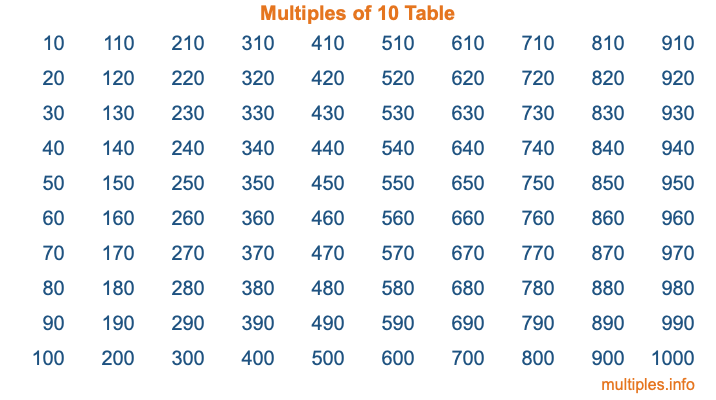Multiples of 10Welcome to the Multiples of 10 page. Here we will first teach you everything you will ever need to know about the multiples of 10, and then give you a study guide summary of everything we taught you to make sure you remember it all. Use this page to look up facts and learn information about the multiples of 10. This page will make you a multiples of ten expert!

Definition of Multiples of 10
Multiples of 10 are all the numbers that when divided by 10 equal an integer. Each of the multiples of 10 are called a multiple. A multiple of 10 is created by multiplying 10 by an integer.

Therefore, to create a list of multiples of 10, you start with 1 multiplied by 10, then 2 multiplied by 10, then 3 multiplied by 10, and so on for as long as you want. Thus, the list of the first five multiples of 10 is 10, 20, 30, 40, and 50. To see a larger list of multiples of 10, see the printable image of Multiples of 10 further down on this page. We also have a category where you can choose any nth multiple of 10.

Multiples of 10 Checker
The Multiples of 10 Checker below checks to see if any number of your choice is a multiple of 10. In other words, it checks to see if there is any number (integer) that when multiplied by 10 will equal your number. To do that, we divide your number by 10. If the the quotient is an integer, then your number is a multiple of 10.

Is  a multiple of 10?

Least Common Multiple of 10 and ...
A Least Common Multiple (LCM) is the lowest multiple that two or more numbers have in common. This is also called the smallest common multiple or lowest common multiple and is useful to know when you are adding our subtracting fractions. Enter one or more numbers below (10 is already entered) to find the LCM.

Check out our LCM Calculator if you need more details about the Least Common Multiple or if you need the LCM for different numbers for adding and subtraction fractions.

nth Multiple of 10
As we stated above, 10 is the first multiple of 10, 20 is the second multiple of 10, 30 is the third multiple of 10, and so on. Enter a number below to find the nth multiple of 10.

th multiple of 10

Multiples of 10 vs Factors of 10
10 is a multiple of 10 and a factor of 10, but that is where the similarities end. All postive multiples of 10 are 10 or greater than 10. All positive factors of 10 are 10 or less than 10.

Below is the beginning list of multiples of 10 and the factors of 10 so you can compare:

Multiples of 10: 10, 20, 30, 40, 50, etc.

Factors of 10: 1, 2, 5, 10

As you can see, the multiples of 10 are all the numbers that you can divide by 10 to get a whole number. The factors of 10, on the other hand, are all the whole numbers that you can multiply by another whole number to get 10.

It's also interesting to note that if a number (x) is a factor of 10, then 10 will also be a multiple of that number (x).

Multiples of 10 vs Divisors of 10
The divisors of 10 are all the integers that 10 can be divided by evenly. Below is a list of the divisors of 10.

Divisors of 10: 1, 2, 5, 10

The interesting thing to note here is that if you take any multiple of 10 and divide it by a divisor of 10, you will see that the quotient is an integer.

Multiples of 10 Table
Below is an image of the first 100 multiples of 10 in a table. The table is in chronological order, column by column. The first column has the first ten multiples of 10, the second column has the next ten multiples of 10, and so on.The Multiples of 10 Table is also referred to as the 10 Times Table or Times Table of 10. You are welcome to print out our table for your studies.

Negative Multiples of 10
Although not often discussed or needed in math, it is worth mentioning that you can make a list of negative multiples of 10 by multiplying 10 by -1, then by -2, then by -3, and so on, to get the following list of negative multiples of 10:

-10, -20, -30, -40, -50, etc.

Multiples of 10 Summary
Below is a summary of important Multiples of 10 facts that we have discussed on this page. To retain the knowledge on this page, we recommend that you read through the summary and explain to yourself or a study partner why they hold true.

There are an infinite number of multiples of 10.

A multiple of 10 divided by 10 will equal a whole number.

10 divided by a factor of 10 equals a divisor of 10.

The nth multiple of 10 is n times 10.

The largest factor of 10 is equal to the first positive multiple of 10.

10 is a multiple of every factor of 10.

10 is a multiple of 10.

A multiple of 10 divided by a divisor of 10 equals an integer.

10 divided by a divisor of 10 equals a factor of 10.

Any integer times 10 will equal a multiple of 10.

Multiples of a Number
Here you can get the multiples of another number, all with the same attention to detail as we did for multiples of 10 on this page.

Multiples of
Multiples of 11
Did you find our page about multiples of ten educational? Do you want more knowledge? Check out the multiples of the next number on our list!